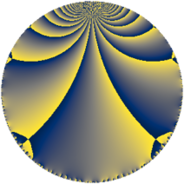Properties

 Label 63.3.qLevel $63$ Weight $3$ Character orbit 63.q Rep. character $\chi_{63}(44,\cdot)$ Character field $\Q(\zeta_{6})$ Dimension $12$ Newform subspaces $1$ Sturm bound $24$ Trace bound $0$

Related objects

Defining parameters

 Level: $$N$$ $$=$$ $$63 = 3^{2} \cdot 7$$ Weight: $$k$$ $$=$$ $$3$$ Character orbit: $$[\chi]$$ $$=$$ 63.q (of order $$6$$ and degree $$2$$) Character conductor: $$\operatorname{cond}(\chi)$$ $$=$$ $$21$$ Character field: $$\Q(\zeta_{6})$$ Newform subspaces: $$1$$ Sturm bound: $$24$$ Trace bound: $$0$$

Dimensions

The following table gives the dimensions of various subspaces of $$M_{3}(63, [\chi])$$.

Total New Old
Modular forms 40 12 28
Cusp forms 24 12 12
Eisenstein series 16 0 16

Trace form

 $$12 q + 16 q^{4} - 2 q^{7} + O(q^{10})$$ $$12 q + 16 q^{4} - 2 q^{7} - 16 q^{10} - 52 q^{13} - 44 q^{16} + 26 q^{19} + 32 q^{22} + 106 q^{25} + 84 q^{28} + 22 q^{31} - 528 q^{34} - 146 q^{37} + 300 q^{40} + 108 q^{43} + 168 q^{46} + 114 q^{49} + 252 q^{52} + 16 q^{55} + 92 q^{58} - 136 q^{61} + 312 q^{64} + 2 q^{67} - 620 q^{70} - 482 q^{73} - 504 q^{76} + 42 q^{79} - 212 q^{82} - 288 q^{85} - 180 q^{88} + 222 q^{91} + 612 q^{94} + 568 q^{97} + O(q^{100})$$

Decomposition of $$S_{3}^{\mathrm{new}}(63, [\chi])$$ into newform subspaces

Label Dim. $$A$$ Field CM Traces $q$-expansion
$a_{2}$ $a_{3}$ $a_{5}$ $a_{7}$
63.3.q.a $12$ $1.717$ $$\mathbb{Q}[x]/(x^{12} - \cdots)$$ None $$0$$ $$0$$ $$0$$ $$-2$$ $$q+\beta _{1}q^{2}+(-3\beta _{8}+\beta _{9})q^{4}-\beta _{6}q^{5}+\cdots$$

Decomposition of $$S_{3}^{\mathrm{old}}(63, [\chi])$$ into lower level spaces

$$S_{3}^{\mathrm{old}}(63, [\chi]) \cong$$ $$S_{3}^{\mathrm{new}}(21, [\chi])$$$$^{\oplus 2}$$